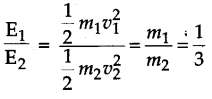# (a) Define work. State two factors on which the magnitude of work depends. (b) A car and a truck have the same speed of 30 m s^-1. If their masses are in the ratio 1:3, find the ratio of kinetic energy?

(a) When an application of force an object gets displaced from its place work is said to be done.
Work depends on force and displacement in the direction of force.
(b) Here, velocity of the two is same, therefore, Ratio of kinetic energy is